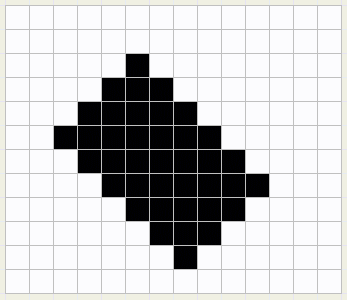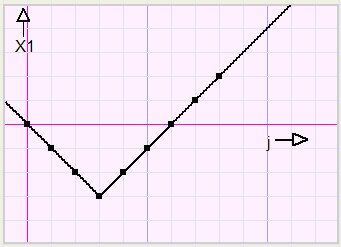Delphi programming techniques the use of "abs" functionsFigure 1 below shows a bitmap with rectangle.
The pixels of this rectangle have to be set to the color black.fig.1
Let us give the top pixel the coordinates (sx,sy).
See figure 2 below. The sides of the rectangle have lengths of h and v.
Say, we are painting the line indicated yellow.
We will accomplish this task by painting a horizontal line from x1 to x2 at y pixels from the top of the bitmap.fig.2
So, the program will look like this:
```var  j : word;
h,v : word;
sx,sy : word;
bm : Tbitmap;
begin
.....
//creation of bitmap
//setting rectangle dimensions h and v
//setting position (sx,sy)
...
with bm.canvas do
begin
pen.color := 0;
pen.width := 1;
for j := 0 to h + v - 2     //from top to bottom of rectangle
begin
y := sy + j;
x1 := ???;
x2 := ???;
moveto(x1,y);
lineto(x2,y)
end;//for j
end;//with bm
....
end;
```
Let's take a look at x1 and it's relation to j.
To simplify the situation we assume that the top pixel has coordinates (0,0).
 j 0 1 2 3 4 5 6 7 8 x1 0 -1 -2 -3 -2 -1 0 1 2
Figure 3 shows the graphicsfig.3
This bended line reminds us of the "absolute" function "abs".fig.4
Above we see the function y = abs(x).

From function theory we know, that if x in a function or equation is replaced by (x-1),
the graph shifts 1 to the right in the coordinate system.
Similar, if y is replaced by (y-1), the graph shifts 1 up
and if y is replaced by (y+1), the graph shifts 1 down.

However, the lowest point is not (0,0) but (3,-3) indicating a right- and downshift of 3.
This changes the function to x1+3 = abs(j-3) or:
x1 = abs(j-3) - 3.

For a height of v the function changes to .............x1 = abs(j+v-1) - (v-1)

Note: moveto(0,0) followed by lineto(10,0) draws a line from (0,0) to (9,0). Pixel (10,0) is not painted.

Finally, sx has to be added, so
x1 = sx + abs(j+v-1) - (v-1)
Similar, the function for x2 is ...............x2 = sx - abs(j-h+1) + (h-1)
The proof is left to the reader.

Note: moveto(0,0) followed by lineto(10,0) draws a line from (0,0) to (9,0). Pixel (10,0) is not painted.
So 1 has to be added to X2 if the lineto(..) method is used.